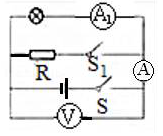$\text{A.}$ 灯L的亮度 $\text{B.}$ 电压表V的示数 $\text{C.}$ 电流表A的示数 $\text{D.}$ 电流表$A_1$的示数